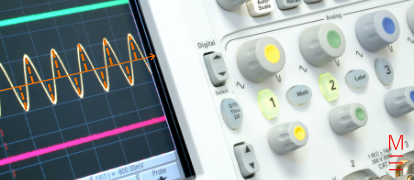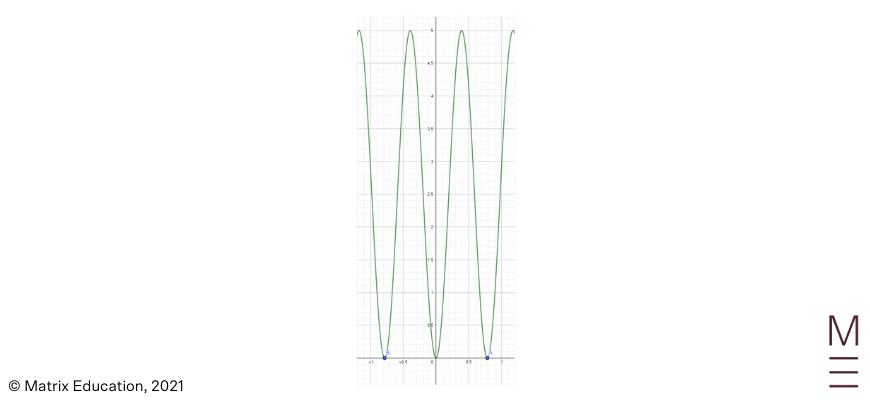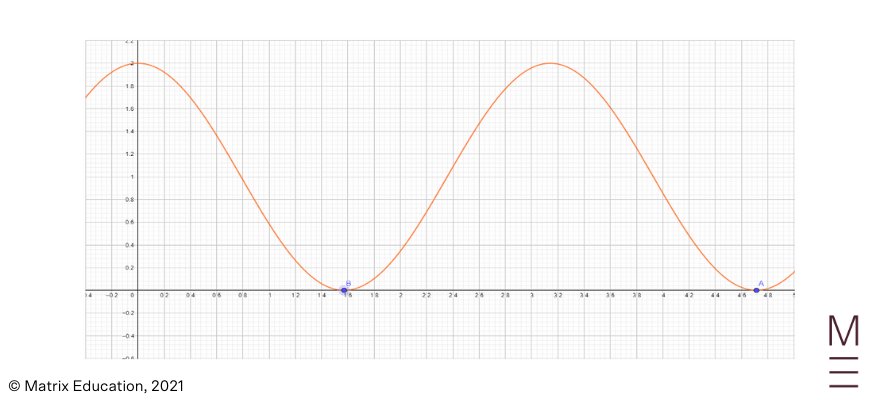# Part 6: Integrating the Squares of Sine and Cosine | Beginner’s Guide to Year 12 Ext 1 Maths

Integrating power and trigonometric functions is very challenging. In this article, we show you how to do it.Integrating power functions and trigonometric functions is very challenging. To help you out,  in this article, we’re going to give you a detailed overview of integrating the squares of sine and cosine.

## Year 12 Mathematics Extension 1: Integration involving $$sin^2(x)$$ and $$cos^2(x)$$

The integration of $$sin^2(x)$$ and $$cos^2(x)$$ may seem daunting at first as it looks like we’re trying to integrate a combination of both a trigonometric and power function. Quickly, we’ll realise that none of our previous approaches to integral calculus appear to make this task simple or straightforward to carry out. However, we can take advantage of certain trigonometric properties to develop a method for tackling these problems.

### NESA Syllabus Outcomes

Students should be proficient in the following outcome after studies in this topic:

• Prove and use the identities $$sin^2nx= \frac{1}{2}(1-cos2nx)$$ and $$cos^2nx=\frac{1}{2}(1+cos2nx)$$ to solve problems
• Solve problems involving $$\int sin^2nx \ dx$$ and $$\int cos^2 nx \ dx$$

## Assumed Knowledge

Students should be familiar with trigonometric identities such as compound and double angle formulas for basic trigonometric ratios. Students should also be comfortable with integral calculus and be able to integrate a variety of functions such as power, trigonometric and exponential functions.

This content can be found in our previous mathematics guides should students want to solidify their understanding.

## Derivation from Compound Angles

Recall that an expression of $$cos(2x)$$ can be derived from the compound angle formula for $$cos(α+β)$$$$=cos(α)⋅cos(β) -sin(α)⋅sin(β)$$. By equation $$α$$ and $$β$$ to $$x$$, we can find that we can rewrite $$cos(2x)=cos^2(x)-sin^2(x)$$.

At this point, you can probably see why this is related to our integration of $$sin^2(x)$$ and $$cos^2(x)$$. To integrate either $$sin^2(x)$$ or $$cos^2(x)$$, we need to isolate our expression above which we can do by applying the Pythagorean trigonometric identity: $$sin^2(x)+cos^2(x)=1$$. To isolate the above expression for $$sin^2(x)$$ we can simply rewrite $$cos(2x)=1-2⋅sin^2(x)$$.

Rearranging,

$$sin^2(x)= \frac{1-cos(2x)}{2}$$

Similarly, if we were to isolate for $$cos^2(x)$$,

$$cos^2(x)= \frac{1+cos(2x)}{2}$$

From our previous study of the integration of trigonometric functions, we can recall that we can integrate trigonometric functions of the linear power, i.e. trig functions that have a power of 1, regardless of the angle inside. We can account for the angle inside by simply applying reverse chain rule and dividing it back. For example, if we consider the following integral:

$$\int cos(ax) \ dx$$

This integral is one of our fundamental results for our integration of trigonometric functions, which we know should simply be $$\frac{1}{a}sin(ax) + C$$.

Combining our rewriting of $$sin^2(x)$$ and $$cos^2(x)$$ in terms of $$cos(2x)$$ as well as our preliminary result of $$\int cos(ax) \ dx=\frac{1}{a}sin(ax)+C$$, we can now determine the integrals of $$sin^2(x)$$ and $$cos^2(x)$$.

## Get more practice with Integration of Sine and Cosine Squared questions!## Integration of $$sin^2(x)$$

From above, we’ve determined that we can rewrite $$sin^2(x)$$ as $$\frac{1-cos(2x)}{2}$$. This means our problem of integrating this function can now be rewritten:

$$\int sin^2(x) \ dx = \int \frac{1-cos(2x)}{2} \ dx$$

This now becomes much easier for us to integrate, as we can simply split the fraction:

\begin{align*}
& \int \left( \frac{1}{2} \ – \frac{1}{2}cos(2x) \right) \ dx\\
&= \frac{1}{2} \int dx \ – \frac{1}{2} \int cos(2x) \ dx\\
&= \frac{1}{2} x \ – \frac{1}{2}⋅\frac{1}{2}sin(2x)+C\\
&= \frac{1}{2}x- \frac{1}{4}sin(2x)+C\\
\end{align*}

This gives us the result of the integral we were looking to find:

$$\int sin^2(x) \ dx = \frac{1}{2} x – \frac{1}{4}sin(2x) + C$$

## Integration of $$cos^2x$$

We can apply a similar methodology to the one we used in evaluating the integral of $$sin^2(x)$$ in solving the integral of $$cos^2(x)$$. We can again take advantage of our expression of $$cos(2x)$$ and rewrite $$cos^2(x)$$ as $$\frac{1+cos(2x)}{2}$$. This simplifies our problem of integrating as we can again rewrite:

$$\int cos^2(x) \ dx = \int \frac{1+cos(2x)}{2} \ dx$$

We then can similarly split the fraction:

\begin{align*}
& \int \left[ \frac{1}{2} + \frac{1}{2}cos(2x) \right] \ dx\\
&= \frac{1}{2} \int dx + \frac{1}{2} \int cos(2x) \ dx\\
&= \frac{1}{2} x + \frac{1}{2} ⋅\frac{1}{2}sin(2x)+C\\
&= \frac{1}{2}x+\frac{1}{4}sin(2x)+C
\end{align*}

This then gives us the following result:

$$\int cos^2(x) \ dx = \frac{1}{2}x + \frac{1}{4}sin(2x)+C$$

## Application to Harder Questions

The above concept is extremely useful in finding the volume generated by a trigonometric curve when it is rotated around an axis.

Below, we will go through some harder and more challenging questions that utilise the identities we have explored and proved above.

### Example 1:

Evaluate the expression $$\int_0^{\frac{π}{4}} \frac{sin2x}{4+cos^2(x)} dx$$, without using integration by substitution.

### Solution:

First, we will first change $$cos^2(x)$$ using the $$cosine$$ double angle formula:

 \begin{align*} cos^2x &= \frac{1+cos(2x)}{2}\\ ∴ \int_0^{\frac{π}{4}} \frac{sin2x}{4+cos^2(x)} dx &= \int_0^{\frac{π}{4}} \frac{sin2x}{4+ \frac{1}{2}(cos2x+1)}dx\\ &= \int_0^{\frac{π}{4}} \frac{sin2x}{\frac{9}{2}+\frac{1}{2}cos2x} dx\\ &= \int_0^{\frac{π}{4}} \frac{2sin2x}{9+cos2x} dx\\ \end{align*}

Now, we can find the integration of this using the integration of logarithms rule:

$$\int \frac{u’}{u} du = log_eu+C$$

However, we should note that the differentiation of $$9+cos2x$$ is $$-2sin2x$$, which we currently do not have exactly. However, we can manipulate our equation like so:

$$=-\int_0^{\frac{π}{4}} \frac{-2sin2x}{9+cos2x} dx$$

Now by applying the logarithms rule, we can easily integrate this normally.

 $$-\int_0^{\frac{π}{4}} \frac{-2sin2x}{9+cos2x} dx = [ -log_e(9+cos2x)]_0^{\frac{π}{4}}$$

To obtain the final answer, we substitute the borders in.

 \begin{align*} [ -log_e(9+cos2x)]_0^{\frac{π}{4}} &= -log_e \left(9+cos \left(2 \times \frac{π}{4} \right) \right)+ log_e(9+cos(0))\\ &= -log_e(9)+log_e(9+1)\\ &= log_e \left( \frac{10}{9} \right) \end{align*}

### Example 2:

Given the curve $$y=5sin^2(4x)$$ below, find the area under the curve from point B to A.The first step in approaching this question is to identify that in order to find the area under the curve, we must use integration. Additionally, we also need to find the coordinates of A and B as they are the borders.Solution:

To find our borders, we need to find the x-intercepts by letting $$y=0$$ then solving for $$x$$.

 \begin{align*} 5sin^2(4x)&=0\\ sin^2(4x) &= 0\\ sin(4x) &= 0\\ 4x&= kπ\\ x &= \frac{kπ}{4} \text{, where k is any integer}\\ B &= \ – \frac{π}{4}, \ \ A = \frac{π}{4} \end{align*}

Now, we can generate an integral to find the area under the curve.

$$A = \int_{- \frac{π}{4}}^{\frac{π}{4}} 5sin^2(4x) \ dx$$

Since our curve is an even function, we can simplify this to:

$$A = 2 \int_0^{\frac{π}{4}} 5 sin^2(4x) \ dx$$

To make the equation a bit simpler, we can also take out any constants.

$$A = 10 \int_0^{\frac{π}{4}} sin^2(4x) \ dx$$

Now, we will change $$sin^2(4x)$$ into something that we can integrate through the cosine double angle formula.

\begin{align*}
A &= 10 \int_0^{\frac{π}{4}} \frac{1-cos(8x)}{2} dx\\
A &= 5 \int_0^{\frac{π}{4}} 1-cos(8x) \ dx\\
A &= 5 \left[ x- \frac{1}{8} sin(8x) \right]_0^{\frac{π}{4}}
\end{align*}

To get rid of the borders, we will substitute them in.

 \begin{align*} A &= 5 \left[ \left( \frac{π}{4} \ – \frac{1}{8}sin \left( 8 \left(\frac{π}{4} \right) \right) \right) – \left( 0 \ – \frac{1}{8}sin(8(0)) \right) \right]\\ &= 5 \left[ \left( \frac{π}{4} \ – \frac{1}{8}sin2π \right) \right] \\ &= 5 \left(\frac{π}{4} \right)\\ &= \frac{5π}{4} u^2 \end{align*}

### Example 3:

Find the volume generated when the curve $$y=1+cos(2x)$$ when the curve is rotated around the x-axis from $$x= \frac{π}{2}$$ to $$x= \frac{3π}{2}$$.### Solution:

The formula for the volume generated when a curve is rotated around the x-axis is given by:

 \begin{align*} V &= \int_b^a y^2 \ dx\\ V &= \int_{\frac{π}{2}}^{\frac{3π}{2}} (1+cos(2x))^2 \ dx\\ &= \int_{\frac{π}{2}}^{\frac{3π}{2}} 1+2cos(2x)+cos^2(2x) \ dx\\ \end{align*}

Again, since we have $$cos^2(2x)$$, we need to utilise the cosine double angle formula to convert this equation into something that we can integrate.

 \begin{align*} V &= \int_{\frac{π}{2}}^{\frac{3π}{2}}  1+2cos(2x) + \frac{1+cos(4x)}{2} \ dx\\ &= \int_{\frac{π}{2}}^{\frac{3π}{2}} \frac{3}{2}+2cos(2x)+ \frac{cos(4x)}{2} \ dx\\ \end{align*}

Now this integral equation is a standard trigonometry integration question, so we can easily find the solution for this.

$$V= \left[ \frac{3}{2}x+sin(2x)+2sin(4x) \right]_{\frac{π}{2}}^{\frac{3π}{2}}$$

By substituting these borders in, we will find the volume of this shape.

 \begin{align*} V &= \left( \frac{3}{2} ( \frac{3π}{2} ) +sin \left( 2 (\frac{3π}{2}) \right) + 2sin \left( 4 ( \frac{3π}{2} ) \right) \right) – \left( \frac{3}{2} ( \frac{π}{2} ) +sin \left( 2 (\frac{π}{2} ) \right) + 2sin \left( 4 (\frac{π}{2}) \right) \right)\\ V &= \left( \frac{9π}{4} +sin(3π)+2sin(6π) \right) – \left( \frac{3π}{4} +sin(π) + 2sin(π) \right)\\ &= \frac{9π}{4} – \frac{3π}{4}\\ &= \frac{6π}{4}\\ &= \frac{3π}{2} u^3\\ \end{align*}

## Concept Check Questions

1. Find the integration of the following equations:

(a) $$y=3sin^2 (\frac{x}{2})$$

(b) $$y= 3 cos^2(3x)$$

(c) $$y= \frac{3}{2}sin^2 ( \frac{5x}{2} )$$

2. Find the area under the curve $$y=4cos^2(2x)$$ from $$x= \frac{π}{4}$$ to $$\frac{3π}{4}$$.

3. Find the area under the curve $$y=3sin^2(7x)$$ from $$x= \frac{π}{7}$$ to $$\frac{2π}{7}$$.

4. What is the volume of the shape generated when the curve $$y=1-sin(x)$$ is rotated around the y-axis from $$x= \frac{π}{2}$$ to $$\frac{5π}{2}$$.

## Concept Check Solutions

1.

(a) $$\frac{3}{2}(x-sinx)+C$$

(b) $$\frac{3}{2} \left( x+ \frac{1}{6} sin(6x) \right) +C$$

(c) $$\frac{3}{4} \left( x- \frac{1}{5}sin(5x) \right)+C$$

2. $$A=π \ u^2$$

3. $$A = \frac{3π}{14} \ u^2$$

4.$$V = 3π \ u^3$$

## Still struggling with Sine and Cosine Squared?

In Matrix+ Online Courses, our HSC experts will guide you through all Maths Ext 1 concepts like Integration Sine and Cosine Squared through clear and structured video lessons. We also provide you with extensive resources that are mailed straight to your doorstep! Learn more now.© Matrix Education and www.matrix.edu.au, 2021. Unauthorised use and/or duplication of this material without express and written permission from this site’s author and/or owner is strictly prohibited. Excerpts and links may be used, provided that full and clear credit is given to Matrix Education and www.matrix.edu.au with appropriate and specific direction to the original content.